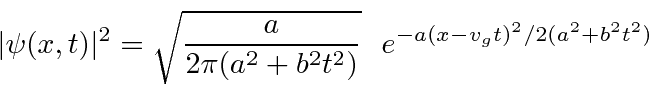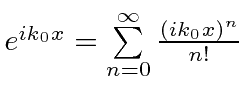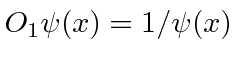## Sample Test Problems

1. The absolute square of a wave function for a free particle is given as:Find the expected value ofas a function of time. Find the expected value ofas a function of time. Compute the RMS x-width of this wave packet as a function of time.
2. Find the commutatorwhereis a constant and the second operator can be expanded as.
3. Which of the following are linear operators?
••••4. For a free particle, the total energy operator H is given by. Compute the commutators [H,x] and [H,p]. If a particle is in a state of definite energy, what do these commutators tell you about how well we know the particle's position and momentum?
5. Find the commutator.
6. Compute the commutatorwhereis the Hamiltonian for a free particle.

Jim Branson 2013-04-22